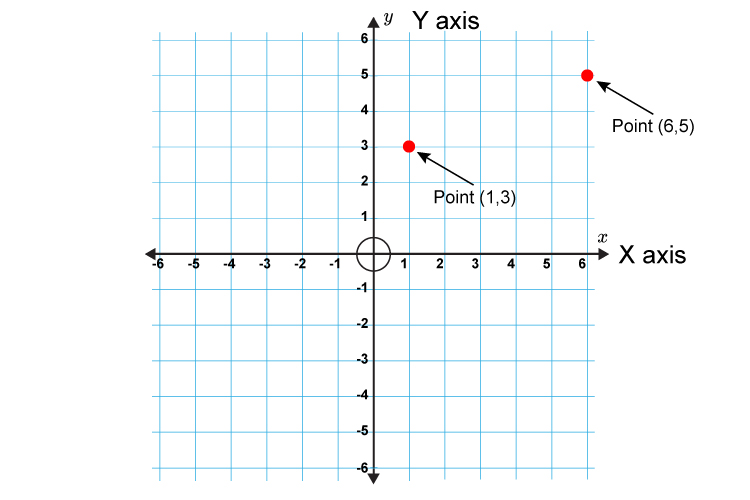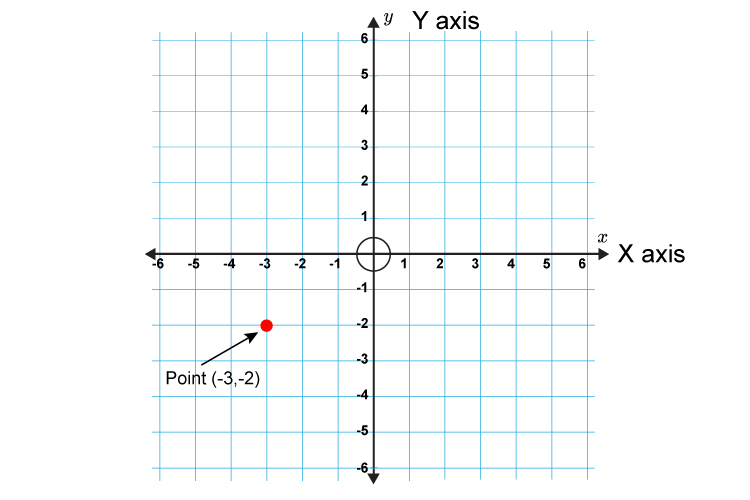# Coordinates – x is first

Coordinates on a graph are written in brackets with a comma in between the x  and the y.

x  is always first.

For example:

(2,3) = 2 along the x  axis and 3 up the y axis.The axe (X-axis) goes first.

Or

Coordinates are written alphabetically
so x comes before y (x,y).

Or

You have to walk across (x) the hall before you can go up (y) the stairs.

Example 1Your starting point is always zero (where the x and the y axis cross). Point (1,3) is a distance of 1 from zero along the x axis and a distance of 3 from zero along the y axis. So point (6,5) is a distance of 6 from zero along the x axis and a distance of 5 from zero along the y axis.

Example 2Point (-3,-2) is a distance of -3 from zero along the xaxis and a distance of -2 from zero along the yaxis.